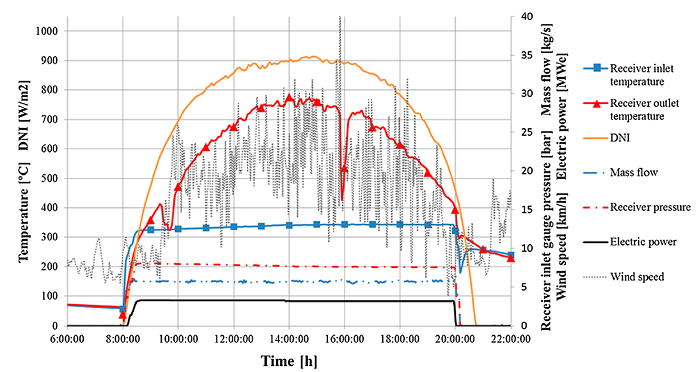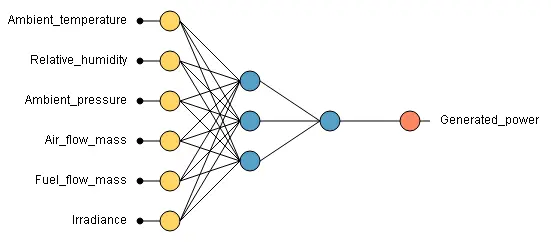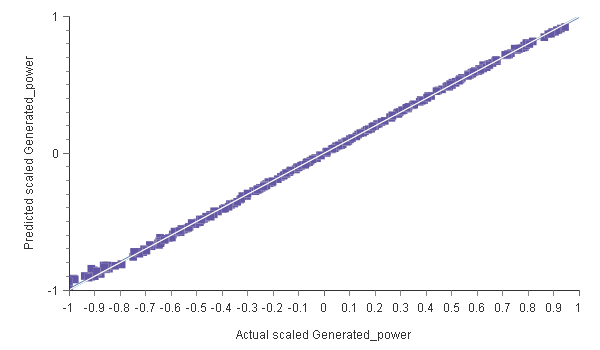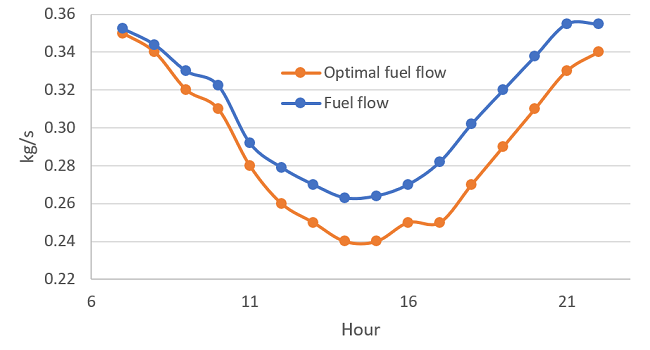In past years, combined cycle power plants have been more used since it has been an increase in their performance concerning the conventional plants.Due to the rapid growth of the energy sector and the electricity demand in the world, a lot of money is being invested in developing new production techniques that support renewable energy and increase its performance against conventional methods.A thermosolar plant consists of a solar-hybrid Brayton cycle that uses concentrated solar power to heat up pressurised air driving a gas turbine.

This is the process that follows a solar-hybrid Brayton plant to produce energy:

• First, the air is collected from the environment by the air source and brought to the compressor. At this point, the air acquires greater pressure, so it also increases its temperature notably.
• Then, the air gets the outflow switch, where the air would choose one way or another depending on the irradiance.
• The next part of the process involves the fuel source and the combustor. In this part, the fluid acquires the temperature and the pressure needed to drive the turbine and the generator.

The objective of this project was to model and then optimise the physical processes that take place in this plant.

First, we needed to construct the data set. Here we used the leading developer of thermal engineering software, called Thermoflex, to collect historical data from all the variables into a single file. As always, the data was treated to fix faults and improve the quality.## Data set

We compared real data obtained from the plant to the one our team calculated using Thermoflex. The next table shows the data obtained from the images, ambient variables for a nominal summer day and the estimated fuel mass flow “Fuel Flow (T)” with its corresponding generated power values “P(T)” so we can compare it to the real ones obtained from thermosolar “(S)”.

Time
(hour)
(W/m2)
Temperature
(K)
Pressure
(bar)
R.H.
(%)
Air Flow
(kg/s)
Fuel flow (S)
(Kg/s)
Power (S)
(MW)
Fuel flow (T)
(Kg/s)
Power (T)
(MW)
7 0 289.079 1.0048 80 17.9 0.353 4.6 0.35 4.827
8 85 289.869 1.0043 80 17.9 0.346 4.6 0.34 4.813
9 240 291.579 1.0047 78 17.9 0.331 4.6 0.33 4.978
22 0 300 1.0046 80 17.9 0.346 4.6 0.35 4.729
As we can see, the fuel values obtained with Thermoflex match with the image of the real data from thermosolar.These are the different variables used in the data set:

• Ambient temperature: This variable describes the ambient temperature so this is a boundary variable. In this case, the unit is Kelvin (K). It has been set as input variable.
• Relative humidity: This variable represents the percentage of humidity in the air so it is also a boundary variable. This variable has been set as input variable.
• Ambient pressure: It represents the ambient pressure in the measure moment so it’s a boundary variable. Its unit is bar (bar) and it has been set as input variable.
• Air flow mass: This variable represents the mass of air which passes per unit of time. It is a control variable and its unit is kg/s.
• Fuel flow mass: This variable represents the mass of fuel which passes per unit of time. It is a control variable and its unit is kg/s.
• Irradiance: The irradiance is the radiant flux (power) received by a surface per unit area. The SI unit of irradiance is the watt per square metre (W/m2). In this example, the irradiance is going to be set as input variable and it is a boundary variable.
• Electrical power: This variable depicts the electrical power output of the combined cycle power plant so this is a state variable. The unit is the mega Watt (MW) and it is the target variable.

## Modelling the physical process

The next step was to study the data and design the neural network. To do that, we applied different statistical methods. Studying the scatter charts, we could clearly see that the generated power increases linearly with the fuel flow mass. This is due to two things:

• On the one hand, the total fluid flow increases, so the power generated also increases its value.
• On the other hand, electricity generation is directly related to the temperature at which the fluid arrives the turbine. Therefore, when more fuel is used in the plant, more temperature acquires the total fluid, and more power generates the plant.

Then, we studied the correlations between the variables and between the variables and the target. The target depends on many inputs simultaneously, but it is interesting to look for linear dependencies among them. You can see the correlations in the following table:

Generated power correlation
Ambient temperature 0.0091
Relative humidity 0.0426
Ambient pressure 0.0102
Air mass flow 0.152
Fuel mass flow 0.974

## Neural networks

A surrogate model is an engineering method used when an outcome of interest cannot be easily directly measured, so a model of the outcome is used instead. Most engineering or physics problems require experiments or simulations to evaluate the objective function and constraint functions as a function of control variables. In this case, the neural network represents our surrogate model.

A graphical representation of the network architecture is depicted in the next picture. It contains a scaling layer, a neural network and an unscaling layer. The yellow circles represent scaling neurons, the blue circles perceptron neurons and the red circles unscaling neurons.After building the neural network, it is necessary to train it to obtain the best possible performance. In this case, we applied the quasi-Newton training method.

We can see that the final selection loss and the final loss are very similar. If these two values were different, it would be producing overfitting during the training.

## Testing of the model

Once the predictive model has been trained, it is necessary to evaluate its predictive power on new data that it has not used before. This will determine how good the model is and determine whether the model is good enough to be moved into the production phase; the grey line would indicate a perfect fit.Another testing method that we can perform is error data statistics. With it, you can measure the minimums, maximums, means and standard deviations of the errors between the neural network and the testing instances in the data set.

As we can see in the previous table, the mean percentage error is 0.54751%, so we can conclude that the model has good accuracy.

Once we have our predictive model tested, we can apply it to predict the generated power by the thermosolar plant whenever we want, depending on the input variables.

## Results

Optimal control is playing an increasingly important role in the design of modern systems. It has as its objective the maximisation of the performance or the minimisation of the costs of production.

Once we have modelled the physical system, in this case, the thermosolar plant production process, we can optimise it by minimising the fuel consumption depending on other variables as irradiance, ambient temperature, ambient pressure, etc.

After defining that our physical constraint will be generated power at 4.6MW, we have to determine which is the optimal (minimum) fuel flow to keep the generated power at the defined level by oscillating the airflow, which is a control variable.

In the following graph, you can observe the optimal fuel that the thermosolar plant should be using and the previous fuel which the plant was using.Using our artificial intelligence technology, the thermosolar plant managed to reduce fuel consumption, which made a big difference in economic savings and improved plant performance.

Concept Amount
Daily fuel consumption (current) 17779 kg/day
Daily fuel consumption (optimal) 16668 kg/day
Yearly fuel savings 405763 kg/year
Fuel price 0.23 €/kg
Yearly money savings 94870 €/year